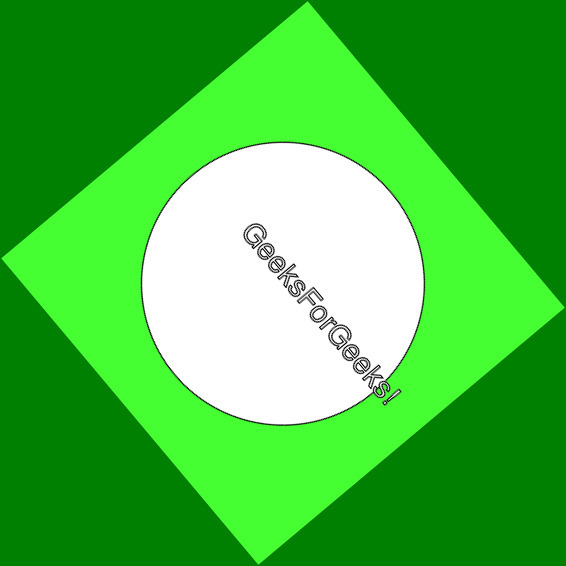Related Articles

# Wand rotate() function – Python

• Last Updated : 04 May, 2020

The rotate() function is an inbuilt function in the Python Wand ImageMagick library which is used to rotates the image right. It takes a background color for which rotation is not available.

Syntax:

`rotate(degree, background, reset_coords)`

Parameters: This function accepts three parameters as mentioned above and defined below:

• degree: This parameter stores the degree of rotation.
• background: This parameter stores the background color for the portion which has no rotation portion available..
• reset_coords: This is a boolean parameter which states after the rotation, the coordinate frame will be relocated to the upper-left corner of the new image. By default is True.

Return Value: This function returns the Wand ImageMagick object.

Original Image:Example 1:

 `# Import library from Image ``from` `wand.image ``import` `Image`` ` `# Import the image``with Image(filename ``=``'../geeksforgeeks.png'``) as image:``    ``# Clone the image in order to process``    ``with image.clone() as rotate:``        ``# Invoke rotate function ``        ``rotate.rotate(``45``, ``'red'``, ``True``)``        ``# Save the image``        ``rotate.save(filename ``=``'rotate1.jpg'``)`

Output:Example 2:

 `# Import libraries from the wand  ``from` `wand.image ``import` `Image``from` `wand.drawing ``import` `Drawing``from` `wand.color ``import` `Color`` ` `with Drawing() as draw:``    ``# Set Stroke color the circle to black``    ``draw.stroke_color ``=` `Color(``'black'``)``    ``# Set Width of the circlw to 2 ``    ``draw.stroke_width ``=` `1``    ``# Set the fill color to 'White (# FFFFFF)'``    ``draw.fill_color ``=` `Color(``'white'``)`` ` `    ``# Invoke Circle function with center at 50, 50 and radius 25``    ``draw.circle((``200``, ``200``), ``# Center point``                ``(``100``, ``100``)) ``# Perimeter point``    ``# Set the font style``    ``draw.font ``=` `'../Helvetica.ttf'``    ``# Set the font size ``    ``draw.font_size ``=` `30``     ` `    ``with Image(width ``=` `400``, height ``=` `400``, background ``=` `Color(``'# 45ff33'``)) as pic:``        ``# Set the text and its location``        ``draw.text(``int``(pic.width ``/` `3``), ``int``(pic.height ``/` `2``), ``'GeeksForGeeks !'``)``        ``# Draw the picture``        ``draw(pic)``        ``# Invoke rotate function method``        ``pic.rotate(``50``, ``'Green'``, ``False``)``        ``# Save the image ``        ``pic.save(filename ``=``'rotate2.jpg'``)`

Output:Attention geek! Strengthen your foundations with the Python Programming Foundation Course and learn the basics.

To begin with, your interview preparations Enhance your Data Structures concepts with the Python DS Course. And to begin with your Machine Learning Journey, join the Machine Learning – Basic Level Course

My Personal Notes arrow_drop_up